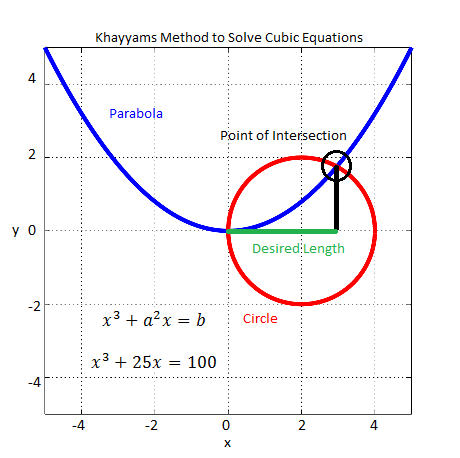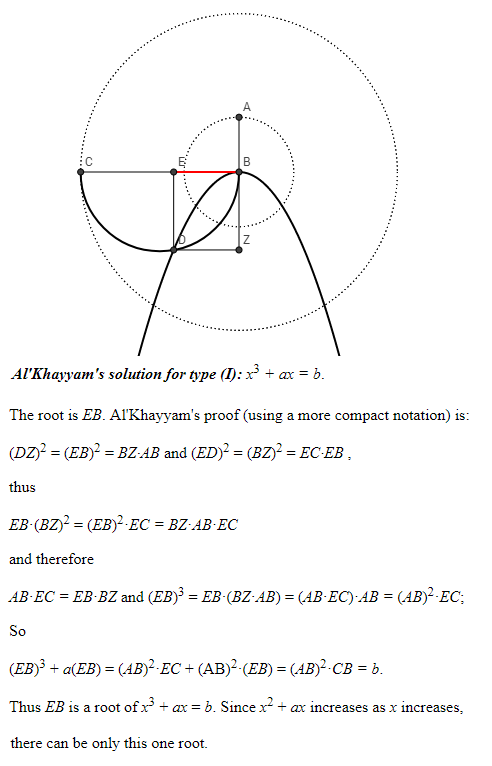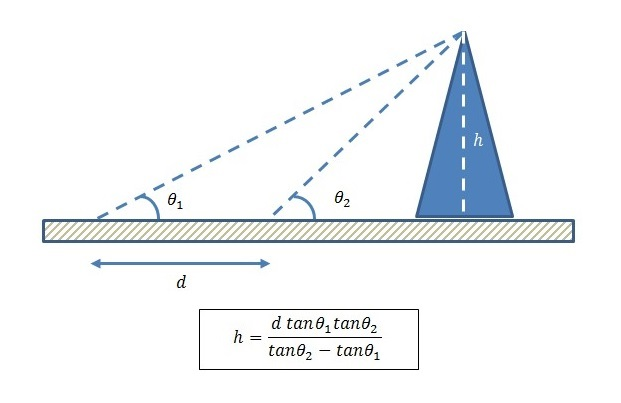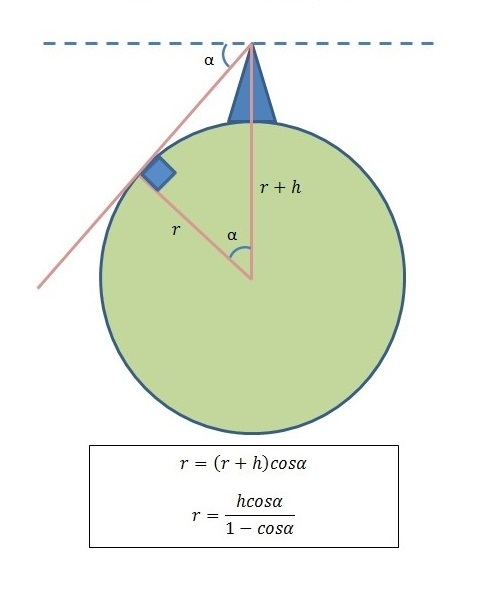# Category Archives: History of Science

The history of science particularly Islamic history.

# My Top 12 Marconi Award Winners

While reading an article on social media I came to know that Siavash M. Alamouti has been awarded the Marconi Award for the year 2022. It came as no surprise as his work on MIMO technology has been ground breaking and has influenced the work of thousands of researchers. If there is a moot point it is that this award must have been given earlier. Just look up his 1998 paper on Google Scholar and you will find that the number of citations has reached a staggering figure of 18,756. On a personal front, I must admit that when I started my research on MIMO I was having difficulty grasping the concepts and it was Alamouti’s paper that set my direction of research.

Continue reading My Top 12 Marconi Award Winners

# Ibn al-Haytham to Maxwell: A Long Road

As the Chinese proverb says “The journey of a thousand miles begins with a single step”. The journey that started with Ibn al-Haytham experimenting with his Camera Obscura in the eleventh century was completed eight hundred years later by James Clerk Maxwell and Heinrich Hertz. While Maxwell laid down the mathematical framework that described the behavior of Electromagnetic waves, Hertz conclusively proved the existing of these invisible waves through his experiments. There were several scientists on the way that played a crucial part in development of this Electromagnetic theory such as Gauss, Faraday and Ampere. Then there were others such as Huygens, Fresnel and Young who worked on nature of light, which was not known to be an Electromagnetic wave at that time. Once the theory  of Electromagnetic wave propagation was in place there was rapid progress in many fields, particularly in wireless communications (wireless telegraph, radio, radar etc.).

Maxwell’s equations that were proposed in 1861 were initially quite circuitous and were not well accepted. But later on these equations were simplified into the form we now know by Oliver Heaviside. There are still two popular forms of the equations, the integral form and the differential form. We present the integral form of these equations in this article as it is more intuitive and is also easier to represent graphically. The differential form requires understanding of the concepts of divergence and curl and we skip it in this article. The main take away from these equations (presented below) is that a changing Electric field produces a Magnetic field and a changing Magnetic field produces an Electric field. Another important result is that magnetic monopoles do not exist (simply put a magnet, however small, always has a north and south pole).

Note:

1. The dot product with a line segment means that only that component of the field vector is effective that is along the line segment. On the other hand the dot product with a surface means that only that component is considered that is perpendicular to the surface (since the unit vector of a surface is perpendicular to the surface). It means that only those field components are considered that are going perpendicularly in or out of the surface.
2. For more on history of Maxwell equations visit IEEE Spectrum  and for a detailed explanation of the various forms of the Maxwell’s equations visit this page.
3. In modern Electromagnetic simulation software the differential form is preferred and the algorithm used is called Finite Difference Time Domain (FDTD). However, if the area of interest is quite large (with respect to the wavelength) then the FDTD method becomes prohibitively complex and another method known as Ray-Tracing is used. Please do check out the Ray-Tracing engine that we have developed. Ray-Tracing is becoming increasingly important in RF Planning of Telecom Networks.

# Omar Khayyam’s Solution to Cubic Equations

Omar Khayyam was a Muslim mathematician and poet of the 11th and 12th centuries (1048-1131). His poetic works known as Rubaiyat of Omar Khayyam were translated from Persian to English and made popular by Edward Fitzgerald in the late nineteenth century. In the field of mathematics his most valuable contribution was the solution he presented to the cubic equations using geometrical methods. Some of this was adapted from earlier works by Greeks but his compilation of the various cases and their solutions was most complete.

Lets assume that the cubic equation also known as the third degree equation (highest power of the unknown variable) is of the form:

x3+a2x=b

Khayyam’s method consisted of constructing a parabola with equation x2=ay and a circle with center (b/2a2,0) and radius b/2a2. Then the x-coordinate of the intersection of the circle and the parabola gives the solution to the cubic equation. The root found by this method is the real and positive root since the length of a line segment cannot be negative or imaginary. These cases (negative and imaginary roots) were not discussed by Khayyam and were worked out much later by other mathematicians. The MATLAB code for this geometrical construction is given below.

```%%%%%%%%%%%%%%%%%%%%%%%%%%%%%%%%
% Omar Khayyams Method to Find
% the Roots of a Cubic Equation
%
%%%%%%%%%%%%%%%%%%%%%%%%%%%%%%%%
clear all
close all

% Plot the parabola
a =5;
x =-5:0.01:5;
y =(x.^2)/a;
plot(x,y,'linewidth',4);
hold on

% Plot the circle
b =100;
d =b/(a.^2);
r =d/2;
t =0:pi/180:2*pi;
plot(r+r*cos(t), r*sin(t),'r', 'linewidth', 4);
hold off
axis([-5 5 -5 5], "square")
grid on
title('Khayyams Method to Solve Cubic Equations')
xlabel('x')
ylabel('y')
```Omar Khayyam’s Method for Solving Cubic Equations

Notes:

1. For more on origins of geometrical methods see the following post on Al-Khwarizmi.
2. For an interactive tool to understand the method of Omar Khayyam visit the following page.
3. For a proof of validity of Khayyam’s method see the following page on Cornell website or see selected abstract below. Please note slightly different form of the equation where the term a2 has been replaced by a. This is just a constant term and either form works.# On the Origins of Snell’s Law (Ibn Sahl’s Law)

Most of intermediate Physics courses present Snell’s law of refraction in one form or another. But a little known mathematician with the name Ibn Sahl (c. 940–1000) found this law about 650 years before Snell (Willebrord Snellius c. 1580–1626). This mathematical expression was lost for centuries until some scholars recently were able to dig it up from historical records. Even Ibn al-Haytham (author of Book of Optics or Kitab ul Manazir) who came to the fore a few years later did not recognize the brilliance of Ibn Sahl’s simple expression.

Ibn Sahl was aware that Greek’s knew that there was a relationship between the angle of incidence and angle of refraction of a ray traveling from one medium to the other. They thought that ratio of the two angles was a constant i.e. if the angle of incidence was doubled the angle of refraction also doubled. This also meant that the arcs formed by the two angles on a circle centered at the point of incidence were also directly related (in a linear relationship). But Ibn Sahl showed that this was incorrect.

Ibn Sahl showed that it was not the angles but the sine of the angles that were linearly related. We explain it with the help of the figure below. Imagine that a ray of light travels from air to a denser medium (such as water), then the ray bends towards the normal and angle of refraction is smaller than angle of incidence. According to Ibn Sahl the ratio of line segments l1 and l2 as shown in the figure is a constant. This in fact means that the two sines have a constant ratio and this is equal to refractive index of the second medium (n2) with refractive index of air almost equal to 1 (n1).

Ibn Sahl was not aware of the parameter ‘n’ defined as refractive index by later scientists. Also, as is known that for small angles, sine of the angle and angle itself are almost the same, so earlier scientists like Ptolemy might have been tricked into assuming that the angles are directly related. This can be understood by looking at the figure above. If the angle of incidence is continuously reduced, the angle of refraction would also decrease and the lengths of the two line segments in red (l1 and l2) would approach the lengths of the arcs that are formed between the ray and the normal.

Note: Roshdi Rashed found the Ibn Sahl text to have been dispersed in manuscripts in two different libraries, one in Tehran, and the other in Damascus. He reassembled the surviving portions, translated and published them as “Geometry and dioptric in the tenth century: Ibn Sahl, al-Quhi and Ibn al-Haytham”.

# Ibn al-Haytham – The Father of Optics

Whenever we read about the history of optics or in general electromagnetics we hear two names again and again, these are James Clerk Maxwell and Heinrich Rudolf Hertz. The discoveries of these two gentlemen although very important to development of Physics were very recent (about 150 years old). But the history of optics is much older and the basics of this field were etched in stone about a 1000 years back. One of the people responsible for the early development of this field was Arab mathematician, astronomer, and physicist ibn al-Haytham (c. 965 – c. 1040).

Ibn al-Haytham in his book referred to as the Book of Optics (Kitāb al-Manāẓir) postulated that light travels in form of rays in straight lines and these rays are reflected by objects which makes these objects visible when the rays enter the human eye. Ibn al-Haytham rejected an earlier theory proposed by Ptolemy and widely accepted by the scholars of that time that light rays emanate from the human eye which makes objects visible. Ibn al-Haytham studied the phenomenon of reflection and refraction of rays of light in his laboratory (he studied lenses, experimented with different mirrors: flat, spherical, parabolic, cylindrical, concave and convex). He also used the Camera Obscura in his experiments to show that light rays travel in straight lines. He studied the working of the human eye and was able to make some valuable contributions.

Other than optics ibn al-Haytham made significant contributions to number theory, geometry, astronomy and natural philosophy. Ibn al-Haytham wrote more than 200 works on a wide range of subjects but most of his works are now lost. Only about 96 are known and about 55 have survived to some extent. Those related to the subject of light include The Light of the Moon, The Light of the Stars, The Rainbow and the Halo, Spherical Burning Mirrors, Parabolic Burning Mirrors, The Burning Sphere, The Shape of the Eclipse, The Formation of Shadows, Discourse on Light, as well as his masterpiece, Book of Optics. It was through Latin and Hebrew translations that most of his important work survived.

UNESCO declared 2015 the International Year of Light and ibn al-Haytham was dubbed as the ‘the father of optics’. This was to celebrate ibn Al-Haytham’s achievements in optics, mathematics and astronomy. In honor of him, the Aga Khan University (Pakistan) named its Ophthalmology endowed chair as “The Ibn-e-Haytham Associate Professor and Chief of Ophthalmology”. The crater Alhazen (latin form of his name) on the Moon is named in his honour, as is the asteroid 59239 Alhazen.

PS: An interesting story about ibn al-Haytham is that he proposed to build a dam on the Nile to restrict the flooding it caused but realized soon that it was impossible to solve this problem with the available resources. Ibn al-Haytham feigned madness so as to not upset the ruler (al-Hakim, the Fatimid caliph in Egypt). Legend has it that he was put into confinement in the city of Cairo near Al Azhar University and it is here that he worked on his theories of optics. Al Azhar University still survives today as one of the greatest and oldest university in the world.

# Al Khwarizmi’s Method of Solving Equations in One Variable

Al Khwarizmi was a Muslim scholar who worked under the patronage of emperor Al Mamun in the 9th century in Baghdad. Al Mamun like his father was interested in supporting learning and formed the House of Wisdom of which Al Khwarizmi was a part. It was here that Greek philosophical and scientific works were translated into Arabic. In recognition of his support Al Khwarizmi dedicated two of his works on algebra and astronomy to the great emperor. His treatise known as Hisab al-jabr w’al-muqabala was the most famous and important one and was translated into several other languages. Over the years al-jabr came to be known as algebra and Al Khwarizmi (Algoritmi  in Latin) became algorithm.

According to Al Khwarizmi equations are linear or quadratic and are composed of units, roots and squares. To Al Khwarizmi a  unit was a number, a root was x and a square was x2. He defined two basic operations to solve equations namely al-jabr (the process of removing negative terms from an equation) and al-muqabala (the process of reducing positive terms of the same power when they occur on both sides of an equation). All Al Khwarizmi mathematics was done entirely in words and no symbol was used e.g. he solves an equation x2+10x=39 as follows:

“… a square and 10 roots are equal to 39 units. The question therefore in this type of equation is about as follows: what is the square which combined with ten of its roots will give a sum total of 39? The manner of solving this type of equation is to take one-half of the roots just mentioned. Now the roots in the problem before us are 10. Therefore take 5, which multiplied by itself gives 25, an amount which you add to 39 giving 64. Having taken then the square root of this which is 8, subtract from it half the roots, 5 leaving 3. The number three therefore represents one root of this square, which itself, of course is 9. Nine therefore gives the square”

One of his masterpieces is his method of solving equations using simple geometry, as shown above. For example to solve the equation x2+10x = 39 he first creates a square with length of each side equal to x and area x2. He then adds 10x to it by creating four rectangles of area 10x/4 = 5x/2 each. Thus the lightly shaded area in figure (c) above represents x2+10x or 39. Now we add the areas of the four corners (darkly shaded) to get  x2+10x+25 or 64 (39+25). So we find out that the side of the larger square is of length 8 (square root of 64). But we already know that the length  of a side is 5/2+x+5/2 = x+5. So we have the final equation x+5 = 8 or x = 3.

# Al Beruni’s Method for Calculation of Radius of Earth

Al Beruni was a great Muslim scientist of the eleventh century who had knowledge of many diverse fields such as astronomy, astrology, mineralogy etc. He was member of Mahmud of Ghazni’s court from 1017 to 1030. It is here that he had the opportunity to travel to India (as well as present day Pakistan) and write about it in his books. It is claimed that he even learned Sanskrit during his stay in India. However, Al Beruni is most well known for his experiment to calculate the radius of the earth at a location close to Katas Raj in Kallar Kahar region of Pakistan. Some claim that the location of his measurements was in fact Nandana fort which is about 40 km east of Katas Raj temples.

His method of calculation involved two steps.

Step 1: Calculate the inclination angle of a mountain at two locations with known separation between them. We can then use simple trigonometry to find the height of the mountain. The trick here is to realize that there are two right angled triangles with the same height, which is an unknown, and base lengths which are also unknown but can be factored out (the reader is encouraged to do the math himself).Figure 1: Calculating the Height of a Mountain

Step 2: The second step involves calculating the angle of depression that the horizon makes as viewed from top of the mountain. Then with the height of the mountain already known the radius of the earth can be easily calculated as shown below.Figure 2: Calculating the Radius of the Earth

As the reader might have noticed there are three angular calculations and one distance calculation involved. Distance is easy to measure but angle is not and an accurate Astrolabe is required for this purpose. The Astrolabe that Al Beruni used was accurate to two decimal places of a degree. The radius that he calculated was within 1% of the accepted radius of the earth today.

PS: The writer has been to Katas Raj temples several times and it his desire to go there again to find more information about the location of the experiment and to possibly re-enact the experiment.

Note:

1. A polymath is a person whose expertise spans a significant number of different subject areas; such a person is known to draw on complex bodies of knowledge to solve specific problems.
2. An Astrolabe is an elaborate inclinometer, historically used by astronomers and navigators to measure the inclined position in the sky of a celestial body, day or night.
3. Nandana was a fort built at strategic location on a hilly range on the eastern flanks of the Salt Range in Punjab Pakistan. Its ruins, including those of a town and a temple, are present. It was ruled by the Hindu Shahi kings until, in the early 11th century, Mahmud of Ghazni expelled them from Nandana.